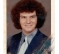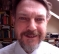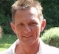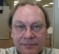## OFF TOPIC: A Question About PIStarted by 4 years ago33 replieslatest reply 4 years ago314 views

Hi. Is the teacher in the following video correct in saying that every number is contained within the digits of pi?

[ - ]A real number that contains any finite sequence of digits appears at least once is called a "rich number".

There are real numbers that have been proven to be rich. Pi isn't one of them.

A stronger criterion is a "normal number", for which any finite sequence of digits appears an infinite number of times, and with the same probability as any other sequence of the same length. All normal numbers are rich (but not the other way around).

There are real numbers that have been proven to be normal. Pi isn't one of them.

Arguments have been put forward for Pi's normality and thus richness, but they are based on empirical observation of the first few billion digits, which is of course 0 percent of the entire sequence.

So, the teacher MAY be correct. We just don't know (yet).

Y(J)S

[ - ]Rick,

The girl in the video asked what pi is good for.  Obviously, it's good for designing the NCO in the demodulator of her smart phone.

[ - ]I agree with LM741 - instead of "number" he meant "integer".  Since Pi isn't randomly distributed, that's kind of mind boggling.  But it must be true of e as well.

[ - ]Dr. Mike! Long time no see (since the 2003 comp.dsp conference)! How are you man?

[ - ]Doing really well - having fun building processor boards.  Not much DSP work, but lots of signal integrity.  Kind of the same thing, just a lot higher frequency :-)  A couple of years ago I had time to post blogs here like Rick, but I don't have time any more.   Nice to see so many people having fun with number theory.  Next step is coding theory.  Then cryptography and elliptic curve math :-)  Number theory is really useful, keep asking questions here!

[ - ]The answer is ‘no’ if  by contains a number you mean that you can find a sequence starting with the first digit of the number and continuing in the same way as the number. Consider an infinite sequence, say the decimal expansion of 1/3. However, it must contain all finite sequences which I think is what is meant in the video.

[ - ]I guess that statement is correct, although very!! irritating.
I enjoyed an old book about a mathematician (Cantor) who was dealing with natural numbers, with alike astonishing proofs. So I learnt to think queer ;-?

Let's assume that pi is contained in pi (but only the digit sequence, without the decimal point, naturally :-)
This is uglier than the original quest because it's an infinite number.
A number with a finite number of digits is certainly easier to prove.
Nevertheless I take it because it makes my approaches easier to explain.

I'll propose two approaches to validate the assumption.

1. First approach:
- find a fraction (with natural numbers) which is smaller than pi ( 9/3)
- find a fraction with the same denominator, but the numerator +1 which is bigger than pi  (10/3)
- now look through pi (beginning from left, if you want)
until you find a sequence which fits into the same limits ( 9/3, 10/3 ). We call it ipi.
- now there's an iteration:
-- double the numbers of the both fractions ( 18/6, 20/6 ). Still pi and the part of pi fits in the new limits
-- now tighten the limits by changing one of the numerators, so that pi is inside the new limits (18/6,19/6)
-- if ipi is out, you got the wrong sequence, so you look through pi until you find a sequence which fits.
-- Cantor proved, that you'll find one ;-)
Since the sequence of digits in pi may not be restricted because it otherwise were a rational number, you'll not find a limit to this iteration, where it fails. So the proof is done :-))
2. Second approach:
- take pi and add ipi, let's say shifted right by 2 digits: (pi + 0.03141...)=>3.173..
-- the exact value is pi*1.01. The error is 1%.
- now comes the iteration:
-- do the same thing but shift by one digit more (3 digits): (pi + 0.003141..) => 3.144734...
-- the exact value is pi*1.001. The error is 0.1%
-- go on with iterations
Since the error will decrease by a factor of 10 with every iteration, the error at infinity is zero.
But if there is not an error anymore, ipi is contained in pi! Q.e.d.

I'm not sure if the second approach satisfies a real mathematician, the first one probably does.

Bernhard
[ - ]Hi bholzmayer. I want to understand your 'First approach'. You wrote,

"...look through pi (beginning from left, if you want)
until you find a sequence which fits into the same limits ( 9/3, 10/3 ). We call it ipi."

Will the number ipi (a number between 3 and 3.3333...) contain a decimal point?

[ - ]Thinking little differently, is there any reason not to believe (at least think think) that irrational numbers exists due to representational aberration. If there is a new number system say “polar numbers” there will be a rational ratio between radius and arc. Is it too much to expect from next generations ? 😉 If the case is possible, then rational numbers can’t hold infinite information.
[ - ]Again, you'll find this question already solved in numbers theory.

The scaling of rational numbers in fact led to the necessary existence of irrational numbers.
Centuries ago, there were famous discussions about the existence of irrational numbers like sqrt(-1).
I guess you'll find the proof in Wikipedia...

[ - ]In English "irrational" means a number that cannot be expressed as a ratio of integers.  sqrt(-1) is called "imaginary", while A + B*sqrt(-1) is called "complex".

(One of my joys in reading Maxwell's "On Governors" for 1868 is that he refers to the roots of a polynomial as containing "impossible parts")

[ - ]sorry, that I had been not precise enough in my "speaking":

Since the quest was that pi contains each number, it would have not been a proof if I would take let's say 97 and show that somewhere within pi you find the digits ...97... Any finite number will certainly appear somewhere, but it would not suffice to prove that. Instead the proof thinks the other way round: If pi contains any number (digit sequence), and hence, if I can prove that the digit sequence of pi itself is contained within pi, then I have proven that all numbers are contained within pi.

Therefore I called ipi a number which is exactly equal pi, but without a decimal point, so just the digit sequence.

Now we take an approach of number theory: every irrational number cannot be represented by a fraction, but will always be "between" the two closest fractions, like pi being between 9/3 and 10/3, but never exact on the fraction.

We are approaching pi by a sequence of fractions, like
(9/3,10/3)
(18/6,19/6)
(37/12,38/12)
...
and we always chose that interval within which the correct pi is found.

In every interval we do the following:

• look for a sequence which starts with the same digits like pi.
example: 3.141592653...
obviously here starts a sequence with the same digit (I don't mind the wrong following digits for the moment). what we do is:
• cut "our" pi before the bold '3' (3.14159265)
• add the sequence 314159265 at the end. => 3.14159265314159265
• check if this number fits within the same interval as pi.
• If yes, go on with the next fraction pair.
If no, you have to look for another sequence which will fit,
you might cut pi at 3.141592653589793... instead, giving
3.14159265358979314159265358979

So, what I called ipi is just the bold part or pi shifted by some digits.

If you think about it, you'll find that it doesn't matter if the following digits after the bold '3' don't fit.
The fractions care about that while we're only going from next to next fraction pair.

Because we're always able to find a sequence which fits within the same interval as pi, there must be a sequence, where the distance between pi and cut(pi)+ipi is smaller than any limit, which means that we finally can say, that the presumption is true, just because we proved that the final error is zero.

Since we made no restrictions to the number of pi except that it is irrational, so we can be sure that the proof holds for every irrational number, like 'e' or sqrt(2).

I'm not a mathematician, and I guess that my proof is not perfect.
But I guess it would be a good starting point for a real proof.

But this has been done centuries ago, and you can find interesting stories laying within this history. Some of those men which dealt with such thoughts too much for a long time, ended brain-damaged (psychotic).
So be cautious ;-)

[ - ]Hi bholzmayer.

Thank you very much for your detailed June 28th "Reply." Number theory is indeed "The Queen of Mathematics."

(And there's no need to warn me about possibly becoming psychotic. According to my family I am already psychotic.)

[ - ]I'm sure you're not in danger to getting psychotic because of touching the area of number theory (otherwise I'd be hospitalized for long ;-).

But if you're trying to find out why there are exact the same number of points on a line within one meter as within one millimeter of it.

Or read about the proof that there exists exactly one rational number for any natural number.

Then you might be interested in Amir D. Aczel's book:
"The mystery of the Aleph: Mathematics, the Kabbalah and the Search for Infinity"

[ - ]The claim

“If pi contains any number (digit sequence), and hence, if I can prove that the digit sequence of pi itself is contained within pi, then I have proven that all numbers are contained within pi.“

is wrong, since there are plenty of number sequences that contain themselves but do not contain all finite numbers, e.g. the decimal expansion of 1/3 does contain itself but only contains digit sequences composed of 3’s.

Looked at another way. First a little lemme, consider any infinite digit sequence s and call the associated number obtained by regarding it as a decimal expansion of the form a.bcd..., #s. Then consider appending some sequence y to s to obtain sy. The number #sy = #s, proof trivial.

Now consider the digit sequence pi and the statement pi contains pi, which is pi = xpiy for some sequence x and y. Now clearly pi is infinite so #piy = #pi, so we need only consider pi = xpi with x a finite prefix. From here we can show #pi is rational since #pi = #x +  10^(-n)*#pi. So (1-10^(-n))*pi = #x, so #pi = 10^n * #x / (10^n - 1) i.e. a rational number, and hence a contradiction.

[ - ]Interesting approach.

1. There's one issue to remember: pi has been proven to be irrational.
You're example with 1/3 deals with a rational number, therefore you have a restricted set of digits, which is not the case for an irrational number.
2. #sy = #s doesn't hold, because adding any value to #s changes it, so it will not be identical. If you talk about the lim(#sy)=lim(#s), I agree.
3. Because of 2, your rationality conclusion does not hold.
You could say that it's close to that rational number (lim), but your simplification goes too far.
4. Interestingly, adding rational numbers, will bring always rational results, but adding any rational number to any irrational number will never bring a rational result.
Example: 1-1/2+1/4-1/8+1/16... must result in a rational number.

[ - ]#4 is quite incorrect as well.  Only true for finite series.  It does not necessarily hold if you get to go to infinity as your ... implies.

Consider the Arctangent Taylor series expression to calculate Pi for a counter example, each term is a rational number.

Pi/4 = 4 * Atn(1 / 5) - Atn(1 / 239)

P.S. jms_nh is right, the topic question is covered in Real Analysis (Ugh indeed).
[ - ]Your claim ‘2’ is wrong - let’s do some aritmetic

x = x + y => y = 0

So obviously

#sy = #s + y’ where y’ is y shifted to the right

#s + y’ = #s => y’ = 0

Unless, of course, you want to claim something special happens with the arithmetic involving irrational numbers

[ - ]I agree, my claim 2 is not correct, because adding the value '0' results in the original value, at least here.
So, I should have noted: "adding any value other than 0".

Which arises another question: is '0' rational or irrational or ... ?
But I guess it's not important here.

[ - ]0 is rational because 0 is an integer and the integers are a subset of the rationals.

Alternatively:

A rational number is a number that can be represented as the ratio of two integers, say a/b.  Since for a=0 and any non-zero b, the value is zero which is then by definition rational.

[ - ]You completely misunderstand the comment - it is not that 0 is a special value that needs to be excluded form your claim. Rather it is that 0 is the only value that y’ can possibly be. hence the implication (=>)

#s + y’ = #s => y’ = 0

[ - ]When I replied earlier I thought I could see the ‘simple’ proof for finite sequences. I was wrong and the more I think about the proof the more I think it is possible but unlikely. If we think of pi as an infinite non-repeating sequence then it is quite possible to have such a sequence that after some initial finite run of using all digits develops a ‘tail’ that omits some particular digit. It can clearly do this and still form a non repeating sequence. And clearly this sequence would not contain numbers containing the omitted digit. So to begin the proof that pi contains all finite numbers we have to start with a proof that pi that no matter how far down pi you go every digit can still occur later in the sequence.

To simplify things it worth moving to the binary expansion where this property is guaranteed. Then the problem becomes one of proving every finite binary sequence is in the binary expansion. Now suppose some n-digit binary sequence is omitted but the first n-1 digits are present, let’s say the omitted sequence ends in 0 and call it s0, then binary pi must contain s1 (the same prefix but ending in 1). I can see absolutely no reason for binary pi not to contain only the s1 form without using some property of pi itself. That is, it is not enough to rely on the ‘infinite nature’ of pi for the same could be said of any irrational

[ - ]Take this challenge: what is the result of the division of  0/0 ?

0? or 1? or 5?

and now, (5*sin(x))/x  or sin(x)/(5x) ?

A theoretical approach uses the derivatives. You may google that...

During my school days (oh boy, that's almost half a century ago ...)
I was fascinated to find out that it really can be everything...

A radial line within a circle. You get the angle with atan(y/x),
for every pair of y/x on the line you get the same angle, so this must be valid even if x->0 and y->0, so atan(0/0) is the angle we had before.
Or any other value for every other angle.

I love that ...

[ - ]Consider this:

The sin(x)/x limit is my example of choice to introduce the concepts of limits to newbies, especially those who struggle with the concept.

Draw a row of circles on a piece of paper.  In the first inscribe a triangle, the second a square, the third a pentagon, and so on.  Now ask the newbie "Would you say as the number of sides increases the length of the perimeter of the inscribed polygons gets closer to the circumference of the circle?"  They will answer in the affirmative, if not, you got a different set of 'splainin' to do.

Then ask them if the following statement makes sense to them:  "The limit as the number of sides approaches infinity of the length of the perimeter is the circumference of the circle"  Say it slowly and clearly and watch for the "aha moment".  Then ask again if it made sense.

Now the concept is grasped, it is time show how it gets expressed mathematically.  So derive the formula for the perimeter using a bisected pie slice, then write the perimeter formula with a leading limit equaling the circumference formula.  Work the algebra until you get to:

lim x->0 [ sin(x)/x ] = 1

Then tell them that that is a very important formula in Mathematics, and especially the math of DSP.  Tell them what you like, but this is what I tell them:

"For DSP, it is called the 'sinc function' and is very important in many roles, including letting you listen to your music.  In Calculus, it is crucial for deriving the derivatives of the trigonometric functions."

Your discussions can go in many directions from there.

Consider also this:

1) Knowing the derivative of the sine is the cosine comes from the sin(x)/x limit

2) Knowing the derivative of the cosine is the negative of the sine also comes from the limit

3) The Taylor series of sine comes from the derivatives of sine and cosine

4) The proof of L'Hopitals Theorem relies on Taylor series

5) L'Hopitals Theorem can be used to prove then sin(x)/x limit.

So, if you rely on the L'Hopitals Theorem to prove the sin(x)/x limit, you really aren't grounded to anything but have a nice dependency ring.

I hope you get a kick out of that.
[ - ]Ugh, a real analysis question. :/

I think it's statistically improbable not to include any finite sequence of digits (in any base, not just decimal). But it's also probably impossible to prove / disprove.

[ - ]But in base pi, pi = 10...

[ - ]I remember that clip from the TV series "Person of Interest". I recall my calculus teacher saying something similar, but more like "every finite number" is contained in every transcendental number (he was trying to impress us). I recall from somewhere else (but not sure where), reading, to the effect that all the words contained in the Library of Congress are in them. Why is that so hard to accept? After all, any finite number should "fit, regardless of how large". It's not that great of a leap of faith, in math, to accept that. Harder to accept is, why is the infinite sum of all positive numbers equal to -1/12? If curious, see: https://www.youtube.com/watch?v=w-I6XTVZXww

[ - ]Hi artmez. Regarding the -1/12 notion, have you seen the analysis at:

[ - ]artmez, ya' know what's weird. The video link I posted contradicts the notion that the sum of all integers is -1/12, but Indian mathematician Srinivasa Ramanujan (whom some people called a "natural mathematical genius") also thought the sum of all integers equals -1/12.

[ - ]Thanks, for that link. It was excellent (he is the most pedantic mathematician I have ever encountered, but I meant that is a good way). I watched it at x1.33 speed to reduce my viewing time from its 41+ minutes. I am more enlightened now but just as flabbergasted as before watching. He did conclude several times that while -1/12 is the answer, that can only be achieved by the additional rules he applied. In the video, he acknowledged that Ramanujan wrote this whole thing in one paragraph, but that the note referenced those additional "adjustments" needed to secure the answer (at 39:16). Still mostly over my head.

Recalling my impression of the original viewing of the Numberphile video, I "thought" that there were some shortcuts being taken, but I didn't remember all the math in my 3rd semester calculus as we covered a lot of material in a short time and then I never really got to use that in my other engineering classes, so I was unsure.

It's reminds me of the often confusing definition of a continuous function, especially when you can do something like a square wave and "know" that it's only continuous if it doesn't have a 0 rise time (i.e. that step point cannot be occupied by two x values), which also makes sense from an engineering perspective, so that it is realizable.

[ - ]Isn't this related to Gödel's incompleteness theorem?

[ - ]